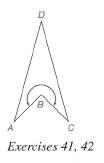Chapter 4.2, Problem 37E### Elementary Geometry for College St...

6th Edition
Daniel C. Alexander + 1 other
ISBN: 9781285195698

#### Solutions

Chapter
Section### Elementary Geometry for College St...

6th Edition
Daniel C. Alexander + 1 other
ISBN: 9781285195698
Textbook Problem
6 views

# In concave kite A B C D , there is an interior angle at vertex B that is a reflex angle. Given that m ∠ A = m ∠ C = m ∠ D = 30 ° , find the measure of the indicated reflex angle.To determine

To find:

The measure of the indicated reflex angle.

Explanation

Calculation:

Given,

A concave kite ABCD

mA=mC=mD=30°

In a kite the sum of all the interior angles is 360°.

Thus,

A+B+C+D=360°

### Still sussing out bartleby?

Check out a sample textbook solution.

See a sample solution

#### The Solution to Your Study Problems

Bartleby provides explanations to thousands of textbook problems written by our experts, many with advanced degrees!

Get Started

#### Find more solutions based on key concepts